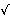There exist triangular numbers that are also squareWonder of wonders. I was very pleasantly surprised by a message from Chris Whitrow:

I just stumbled across your site and noticed your page on triangular and square numbers. I couldn't help dropping this note to point out the curious fact that there is also an infinite set of numbers which are simultaneously both triangular and square. There is a recurrence relation for generating them:

 (1) S(n+1) = 4·S(n)·[8·S(n) + 1]

Starting with S(1) = 1, S(2) = 36, and so on. Proof is fairly straightforward. Well, it seems curious that a thing can be both a triangle and a square, doesn't it?

Yes, of course. How can something be square and triangular simultaneously?

Well, I had to look through several books in my library till I discovered a discussion of these numbers in A. H. Beiler's Recreations in the Theory of Numbers. Beiler writes: "Eight triangles increased by unity produce a square," because

 (2) 8·r(r + 1)/2 + 1 = 4r2 + 4r + 1 = (2r + 1)2.

(2) proves the converse as well: a square decreased by unity gives eight triangles. This simple rule explains the recurrence relation (1). However, (1) does not enumerate all such numbers. From (1), we get S(3) = 41616, whereas there is another number, 1225, that is both triangular and square: 1225 = 49·50/2 = 352.

The question was in fact posted as the problem E1473 (AMM, 1962, p. 168) by J.L. Pietepol and solved by A.V. Sylvester [Mathematical Morsels, p. 145, Mathematical Quickies, #186]. Let Tn = n(n + 1)/2 denote the nth triangular number. The solution follows from the observation that if Tn is a perfect square, so is T4n(n+1). Indeed, let n(n + 1)/2 = k2. Then 4n(n + 1) = 8k2. Continue:

 T4n(n+1) = T8k2 = 8k2(8k2 + 1)/2 = 4k2(8k2 + 1) = 4k2[4n(n + 1) + 1] = 4k2[4n2 + 4n + 1] = 4k2(2n + 1)2.

All such numbers can be found by solving a Pell equation: 8x2 + 1 = y2 which plays a prominent role in number theory.

Numbers Simultaneously Square and Triangular
8x2 + 1 = y2Triangular numberSide of triangleSide of square
8·12+1 = 32111
8·62+1 = 1723686
8·352+1 = 99212254935
8·2042+1 = 577241616288204
8·11892+1 = 33632141372116811189
8·69302+1 = 1960124802490098006930
8·403912+1 = 114243216314328815712140391

All such numbers can be obtained by squaring the members of the sequence generated from the recurrence relation

 (3) un = 6un-1 - un-2, n > 1, u0 = 0, u1 = 1,

There is also an explicit formula

 (4) un = (((1 + √2)2n - (1 - √2)2n)/(42))2

which can also be written as [Wells, p. 93]

 (4) un = [(17 + 12√2)n + (17 - 12√2)n - 2]/32.

([Wells, p. 93] also mentions that no triangular number may be a cube, or fourth or fifth power.)

Armando Guarnaschelli from Argentina found a very simple recurrence relation that generates all triangular numbers that are also square.

References I came across are listed below.

### References

1. A. H. Beiler's Recreations in the Theory of Numbers, Dover, 1966
2. J. H. Conway, R. K. Guy, The Book of Numbers, Springer, 1996
3. R. Honsberger's Mathematical Morsels, MAA, 1978
4. G. M. Phillips, Mathematics Is Not a Spectator Sport, Springer, 2005
5. C. W. Trigg, Mathematical Quickies, Dover, 1985
6. D. Wells, The Penguin Dictionary of Curious and Interesting Numbers, Penguin Books, 1987